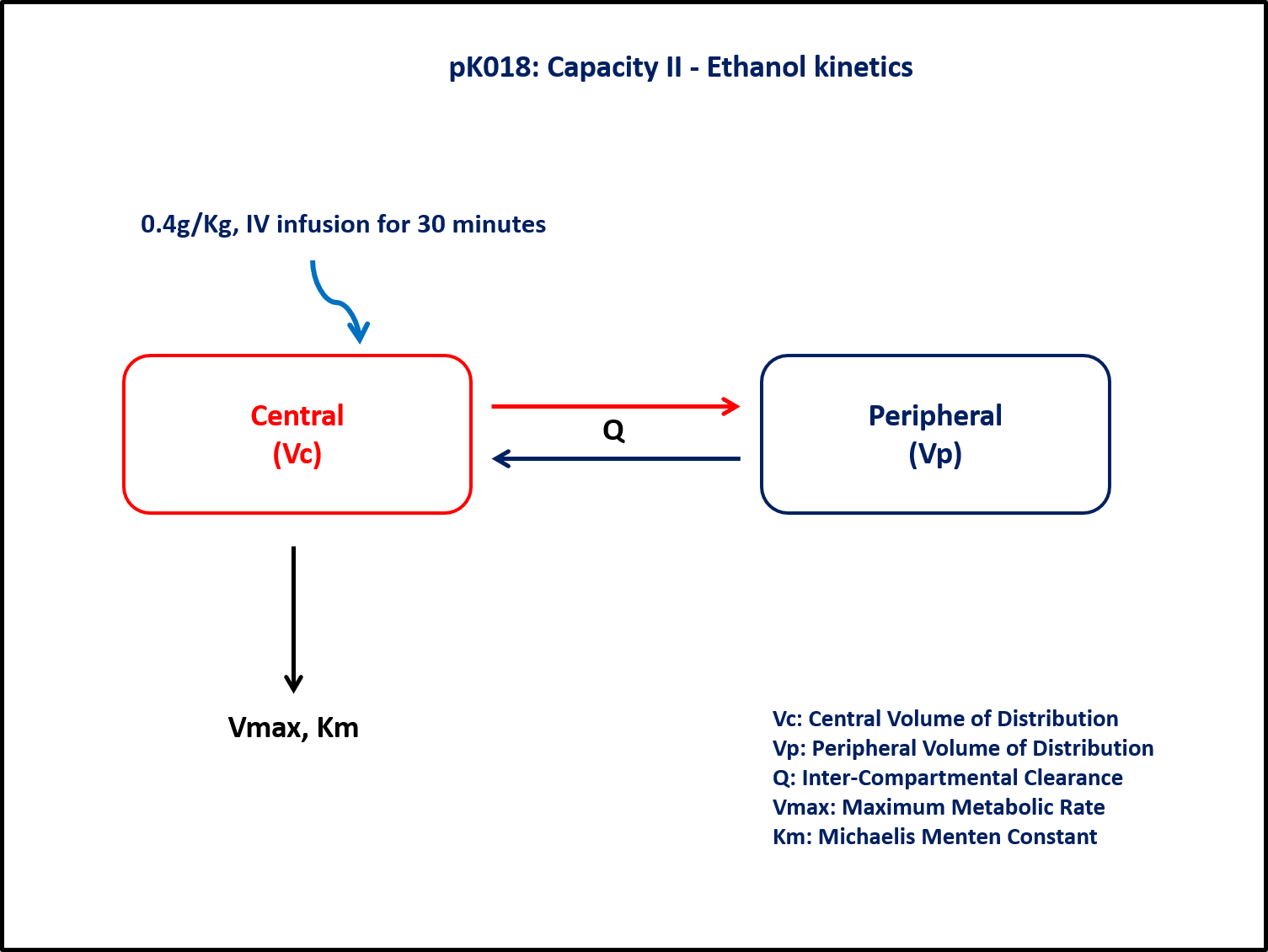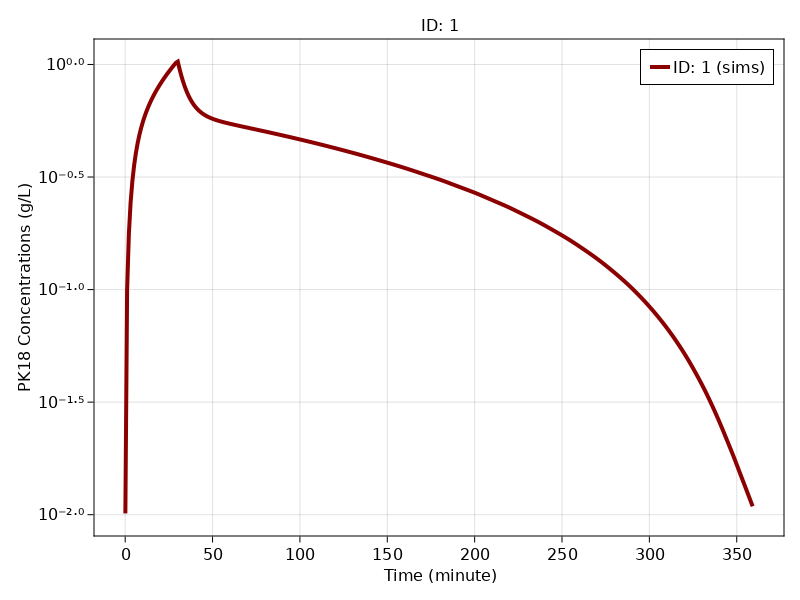# Exercise PK18 - Capacity II - Ethanol kinetics

### Background

• Structural model - Two compartment disposition model with nonlinear elimination

• Route of administration - IV infusion

• Dosage Regimen - 0.4g/Kg (i.e.,28g for a 70Kg healthy individual), infused over a time span of 30 minutes

• Number of Subjects - 1### Learning Outcome

In this model, you will learn -

• To build a two compartment disposition model, the drug is given as an Intravenous Infusion which follows Michaelis-Menten Kinetics.

• To apply differential equation in the model as per the compartment model.

• To design the dosage regimen for the subjects and simulate the plot.

### Objectives

In this tutorial, you will learn how to build a two Compartment disposition model with Non-linear elimination following Intravenous infusion and simulate the model for single subject and single dosage regimen.

### Libraries

Call the "necessary" libraries to get started

using Random
using Pumas
using PumasUtilities
using CairoMakie


### Model

In this two compartment model, we administer dose on Central compartment.

pk_18           = @model  begin
desc = "Two Compartment Model - Nonlinear Elimination"
timeu = u"hr"
end

@param begin
"Maximum rate of elimination (mg/hr)"
tvvmax      ∈ RealDomain(lower=0)
"Michaelis-Menten rate constant (mg/L)"
tvkm        ∈ RealDomain(lower=0)
"Intercompartmental Clearance (L/hr)"
tvQ         ∈ RealDomain(lower=0)
"Volume of Central Compartment (L)"
tvvc        ∈ RealDomain(lower=0)
"Volume of Peripheral Compartment (L)"
tvvp        ∈ RealDomain(lower=0)
Ω           ∈ PDiagDomain(5)
"Proportional RUV"
σ_prop     ∈ RealDomain(lower=0)
end

@random begin
η           ~ MvNormal(Ω)
end

@pre begin
Vmax        = tvvmax * exp(η)
Km          = tvkm * exp(η)
Q           = tvQ * exp(η)
Vc          = tvvc * exp(η)
Vp          = tvvp * exp(η)
end

@dynamics begin
Central'    = - (Vmax/(Km+(Central/Vc))) * Central/Vc + (Q/Vp) * Peripheral - (Q/Vc) * Central
Peripheral' = - (Q/Vp) * Peripheral + (Q/Vc) * Central
end

@derived begin
cp         = @. Central/Vc
"""
Observed Concentration (g/L)
"""
dv         ~ @. Normal(cp, cp^2*σ_prop)
end
end

PumasModel
Parameters: tvvmax, tvkm, tvQ, tvvc, tvvp, Ω, σ_prop
Random effects: η
Covariates:
Dynamical variables: Central, Peripheral
Derived: cp, dv
Observed: cp, dv


### Parameters

The parameters are as given below. tv represents the typical value for parameters.

• $Vmax$ - Maximum rate of elimination (mg/hr)

• $Km$ - Michaelis-Menten rate constant (mg/L)

• $Q$ - Intercompartmental Clearance (L/hr)

• $Vc$ - Volume of Central Compartment (L)

• $Vp$ - Volume of Peripheral Compartment (L)

• $Ω$ - Between Subject Variability

• $σ$ - Residual error

param  = (tvvmax     = 0.0812189,
tvkm       = 0.0125445,
tvQ        = 1.29034,
tvvc       = 8.93016,
tvvp       = 31.1174,
Ω          = Diagonal([0.0,0.0,0.0,0.0,0.0]),
σ_prop    = 0.005)

(tvvmax = 0.0812189, tvkm = 0.0125445, tvQ = 1.29034, tvvc = 8.93016, tvvp
= 31.1174, Ω = [0.0 0.0 … 0.0 0.0; 0.0 0.0 … 0.0 0.0; … ; 0.0 0.0 … 0.0 0.0
; 0.0 0.0 … 0.0 0.0], σ_prop = 0.005)


### Dosage Regimen

0.4g/Kg (i.e.,28g for a 70Kg healthy individual), infused over a time span of 30 minutes, given to a single subject.

ev1   = DosageRegimen(28, time = 0, cmt = 1, duration = 30)
sub1  = Subject(id = 1, events = ev1, observations = (cp = nothing,))

Subject
ID: 1
Events: 2
Observations: cp: (nothing)


### Simulation

Lets simulate for plasma concentration with the specific observation time points after Intravenous administration.

Random.seed!(1234)
sim_sub = simobs(pk_18,sub1, param, obstimes = 0.1:1:360)


### Visualization

f, a, p = sim_plot(pk_18, [sim_sub],
observations = :cp,
color = :redsblues,
linewidth = 4,
axis = (xlabel = "Time (minute)",
ylabel = "PK18 Concentrations (g/L)",
xticks = 0:50:400, yscale = log10))
axislegend(a)
f